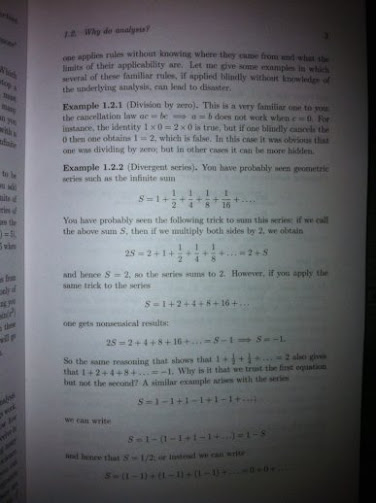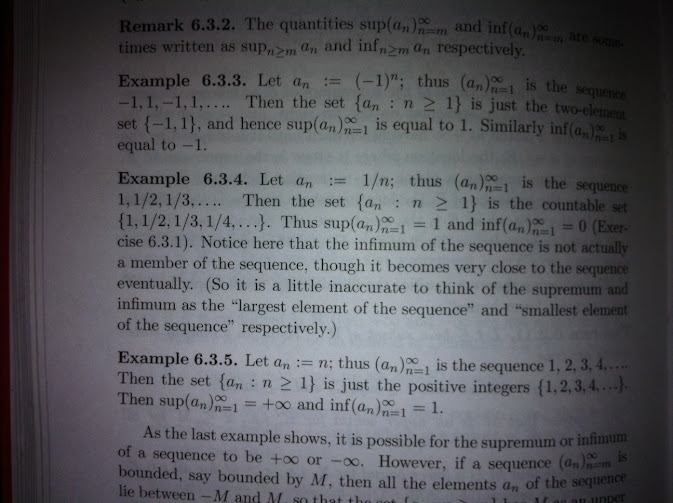# Summing divergent series based on physics

Solving a heat equation with central symmetry i got the following result:

The problem is to find a sphere center temperature vs. time given that the surface of the sphere is kept constant at $$T_1$$ and at initial moment t=0 the temperature inside the sphere is distributed uniformly with value $$T_0$$

A solution is following:

$$T(t)=T_1+2 (T_0-T_1)\sum_{k=1}^\infty (-1)^{k+1} e^{-t(\frac{k\pi a}{r})^2}$$ Here r is the radius of the sphere and a=const is a coefficient of thermal conductivity.

Now the solution must satisfy the initial condition $$T(0)=T_0$$:

$$T(0)=T_1+2 (T_0-T_1)\sum_{k=1}^\infty (-1)^{k+1}$$

This is satisfied only if the following holds:

1-1+1-1+1-1+ ... =1/2

So, we found this divergent sum through physical arguments? Is this appropriate for the pure mathematician?

Lets go a step further and differentiate the solution by t:

$$\frac {d T(t)}{dt}=-2 (T_0-T_1)(\frac{\pi a}{r})^2\sum_{k=1}^\infty (-1)^{k+1} k^2 e^{-t(\frac{k\pi a}{r})^2}$$

Now, at initial moment t=0 we have:

$$\frac {d T(0)}{dt}=-2 (T_0-T_1)(\frac{\pi a}{r})^2\sum_{k=1}^\infty (-1)^{k+1} k^2$$

Obviously $$\frac {d T(0)}{dt}=0$$ because the maximum speed in the universe is limited.

Thus the following must be valid:

1^2 - 2^2 + 3^2 - 4^2 + ... =0

Here we can say what has been said previously, i think. But at the moment i'm not sure about the following divergent sum:

1 - 2 + 3 - 4 + ...

• Have you attempted constructing a Padé approximant from your formally divergent series? That's one thing they're useful for. – J. M. isn't a mathematician Nov 12 '10 at 12:31
• As a matter of fact, you ought to look at convergence acceleration techniques in general; they work surprisingly well for extracting useful information from divergent series. Something like $1+2+4+8+16+\dots=-1$ for instance. – J. M. isn't a mathematician Nov 12 '10 at 12:35
• Also this post of Terry Tao on divergent sums might be useful. – Marek Nov 12 '10 at 15:57

(Background for other readers: the derivation of the solution can be found on Google books, see equations (6.18) and (6.19).)

This is a good and very natural question, but your reasoning is flawed.

The solution formula that you quote for $T(t)$ is only valid for $t>0$, and it does satisfy $\lim_{t\to 0} T(t) = T_0$; it's just that taking the limit $t\to 0$ termwise isn't justified.

(That the limit is $T_0$ can be seen by tracing all the steps in the derivation carefully and using knows facts about convergence of Fourier series. Unfortunately, explaining this would require drawing more figures than I can do here with a reasonable amount of effort... Someone who knows more than I about theta functions can probably say something about how to compute the limit directly.)

Your argument "because the maximum speed in the universe is limited" is useless, since the heat equation is not the real world; in fact the heat equation admits infinite speed of propagation! (A solution with compact support at $t=0$ will not have compact support for any $t>0$.) Moreover, taking the limit $t\to 0$ termwise is even less justified in the differentiated sum than it was in the original sum.

It was good enough for Euler and Ramanujan apparently.

1-2+3-4+...

1+2+3+4+...

The following literature may be of use here:SOURCE: "Analysis I" (second edition) by Terence Tao